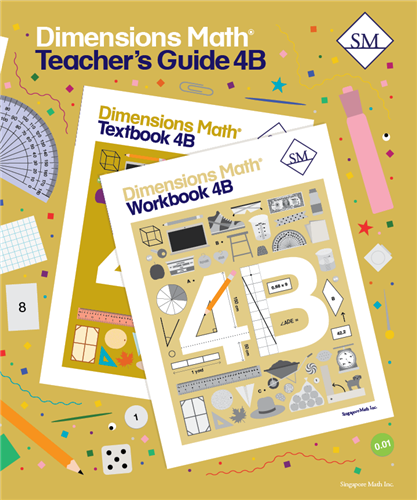# Dimensions Math Teacher's Guide 4B

\$ 25.00

Dimensions Math® Teacher's Guide 4B

Chapter 10: Measurement
Teaching Notes
Chapter Opener
Lesson 1: Metric Units of Measurement
Lesson 2: Customary Units of Length
Lesson 3: Customary Units of Weight
Lesson 4: Customary Units of Capacity
Lesson 5: Units of Time
Lesson 6: Practice A
Lesson 7: Fractions of Measurement — Part 1
Lesson 8: Fractions of Measurement — Part 2
Lesson 9: Practice B
Workbook Solutions

Chapter 11: Area and Perimeter
Teaching Notes
Chapter Opener
Lesson 1: Area of Rectangles Part 1
Lesson 2: Area of Rectangles Part 2
Lesson 3: Area of Composite Figures
Lesson 4: Perimeter — Part 1
Lesson 5: Perimeter — Part 2
Lesson 6: Practice
Workbook Solutions

Chapter 12: Decimals
Teaching Notes
Chapter Opener
Lesson 1: Tenths — Part 1
Lesson 2: Tenths — Part 2
Lesson 3: Hundredths — Part 1
Lesson 4: Hundredths — Part 2
Lesson 5: Expressing Decimals as Fractions in Simplest Form
Lesson 6: Expressing Fractions as Decimals
Lesson 7: Practice A
Lesson 8: Comparing and Ordering Decimals
Lesson 9: Rounding Decimals
Lesson 10: Practice B
Workbook Solutions

Chapter 13: Addition and Subtraction of Decimals
Teaching Notes
Chapter Opener
Lesson 1: Adding and Subtracting Tenths
Lesson 2: Adding Tenths with Regrouping
Lesson 3: Subtracting Tenths with Regrouping
Lesson 4: Practice A
Lesson 6: Subtracting from 1 and 0.1
Lesson 7: Subtracting Hundredths
Lesson 8: Money, Decimals, and Fractions
Lesson 9: Practice B
Review 3
Workbook Solutions

Chapter 14: Multiplication and Division of Decimals
Teaching Notes
Chapter Opener
Lesson 1: Multiplying Tenths and Hundredths
Lesson 2: Multiplying Decimals by a Whole Number — Part 1
Lesson 3: Multiplying Decimals by a Whole Number — Part 2
Lesson 4: Practice A
Lesson 5: Dividing Tenths and Hundredths
Lesson 6: Dividing Decimals by a Whole Number — Part 1
Lesson 7: Dividing Decimals by a Whole Number — Part 2
Lesson 8: Dividing Decimals by a Whole Number — Part 3
Lesson 9: Practice B
Workbook Solutions

Chapter 15: Angles
Teaching Notes
Chapter Opener
Lesson 1: The Size of Angles
Lesson 2: Measuring Angles
Lesson 3: Drawing Angles
Lesson 4: Adding and Subtracting Angles
Lesson 5: Reflex Angles
Lesson 6: Practice
Workbook Solutions

Chapter 16: Lines and Shapes
Teaching Notes
Chapter Opener
Lesson 1: Perpendicular Lines
Lesson 2: Parallel Lines
Lesson 3: Drawing Perpendicular and Parallel Lines
Lesson 5: Lines of Symmetry
Lesson 6: Symmetrical Figures and Patterns
Lesson 7: Practice
Workbook Solutions

Chapter 17: Properties of Cuboids
Teaching Notes
Chapter Opener
Lesson 1: Cuboids
Lesson 2: Nets of Cuboids
Lesson 3: Faces and Edges of Cuboids
Lesson 4: Practice
Review 4
Review 5
Workbook Solutions

Dimensions Math® PreK-5 series features the progression, rigor, and pacing that define Singapore math. Throughout the series, five characters offer students suggestions on how to think about problems. They remind students of strategies they’ve learned and point out important information that encourages them to come up with their own solutions.

Textbook lessons begin with a task that allows students to apply their previous knowledge and learn through discussion. Once students have mastered a concept with the use of concrete and pictorial aids, they are ready to take on more abstract mathematical problem sets. They reach fluency by collecting various strategies along the way and applying them to new problems. Word problems give students a sense of math in real-world contexts.

Workbooks offer independent practice that follows a careful progression of exercise variation. Each textbook lesson includes a corresponding workbook exercise that starts with pictorial representation and progresses to more challenging abstract problems. Workbooks for PreK-2 are perforated.

Teacher’s Guides include lesson plans, mathematical background, games, helpful suggestions, and comprehensive resources for daily lessons. Lessons are laid out clearly and activities are designed for the whole class, small groups, and extension.

Textbooks and Workbooks do not include answer keys. Answers are in Teacher's Guides.
ISBN  9781947226395